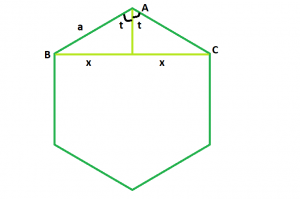Length of Diagonal of a n-sided regular polygon

• Last Updated : 17 Mar, 2021

Given a n-sided regular polygon of side length a.The task is to find the length of it’s diagonal.
Examples:

Input:  a = 9, n = 10
Output:  17.119

Input: a = 4, n = 5
Output: 6.47213Approach:

We know that the sum of interior angles of a polygon = (n – 2) * 180 where, n is the no. of sides in the polygon.
So, each interior angle = (n – 2) * 180/n
Now, we have to find BC = 2 * x. If we draw a perpendicular AO on BC, we will see that the perpendicular bisects BC in BO and OC, as triangles AOB and AOC are congruent to each other.
Now, t = (n – 2) * 180/2n
So, sint = x/a
Therefore, x = asint
Hence, diagonal=2x = 2asint = 2asin((n – 2) * 180/2n)

C++

 // C++ Program to find the diagonal// of a regular polygon with given side length#include using namespace std; // Function to find the diagonal// of a regular polygonfloat polydiagonal(float n, float a){     // Side and side length cannot be negative    if (a < 0 && n < 0)        return -1;     // diagonal    // degree converted to radians    return 2 * a * sin((((n - 2) * 180) / (2 * n)) * 3.14159 / 180);} // Driver codeint main(){    float a = 9, n = 10;    cout << polydiagonal(n, a) << endl;     return 0;}

Java

 // Java Program to find the diagonal// of a regular polygon with given side length class GFG { // Function to find the diagonal// of a regular polygon    static float polydiagonal(float n, float a) {         // Side and side length cannot be negative        if (a < 0 && n < 0) {            return -1;        }         // diagonal        // degree converted to radians        return (float) (2 * a * Math.sin((((n - 2) * 180) / (2 * n)) * 3.14159 / 180));    } // Driver code    public static void main(String[] args) {        float a = 9, n = 10;        System.out.printf("%.3f",polydiagonal(n, a));     }} // This code is contributed by 29AjayKumar

Python3

 # Python3 Program to find the diagonal# of a regular polygon with given side lengthimport math as mt # Function to find the diagonal# of a regular polygondef polydiagonal(n, a):     # Side and side length cannot    # be negative    if (a < 0 and n < 0):        return -1     # diagonal degree converted to radians    return (2 * a * mt.sin((((n - 2) * 180) /           (2 * n)) * 3.14159 / 180)) # Driver codea, n = 9, 10print(polydiagonal(n, a)) # This code is contributed# by Mohit kumar 29

C#

 // C#  Program to find the diagonal// of a regular polygon with given side lengthusing System; public class GFG{     // Function to find the diagonal// of a regular polygon    static float polydiagonal(float n, float a) {         // Side and side length cannot be negative        if (a < 0 && n < 0) {            return -1;        }         // diagonal        // degree converted to radians        return (float) (2 * a * Math.Sin((((n - 2) * 180) / (2 * n)) * 3.14159 / 180));    } // Driver code    static public void Main (){            float a = 9, n = 10;        Console.WriteLine(polydiagonal(n, a));     }} // This code is contributed by  @Sachin...



Javascript


Output:
17.119

My Personal Notes arrow_drop_up